# The Calculator Encyclopedia Performs Calculations on Binomial Series

According to Wikipedia,

In mathematics, the binomial series is the Maclaurin series for the function given by , where is an arbitrary complex number. Explicitly, and the binomial series is the power series on the right hand side of (1), expressed in terms of the (generalized) binomial coefficients.

Nickzom Calculator performs calculations on Binomial Series for both addition and subtraction.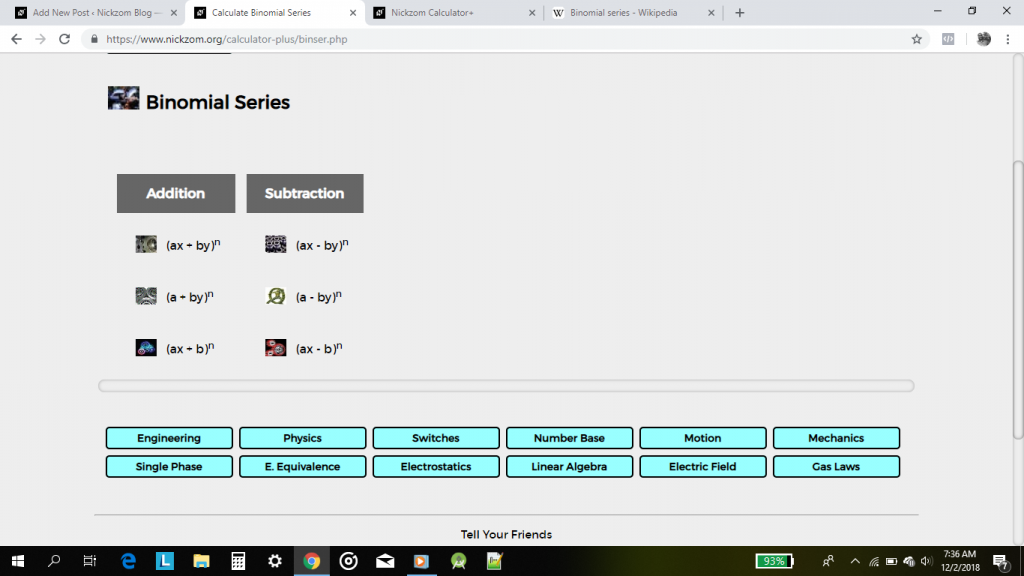If you have been following this blog for a while, you would know that the first thing is to proceed to the Calculator Map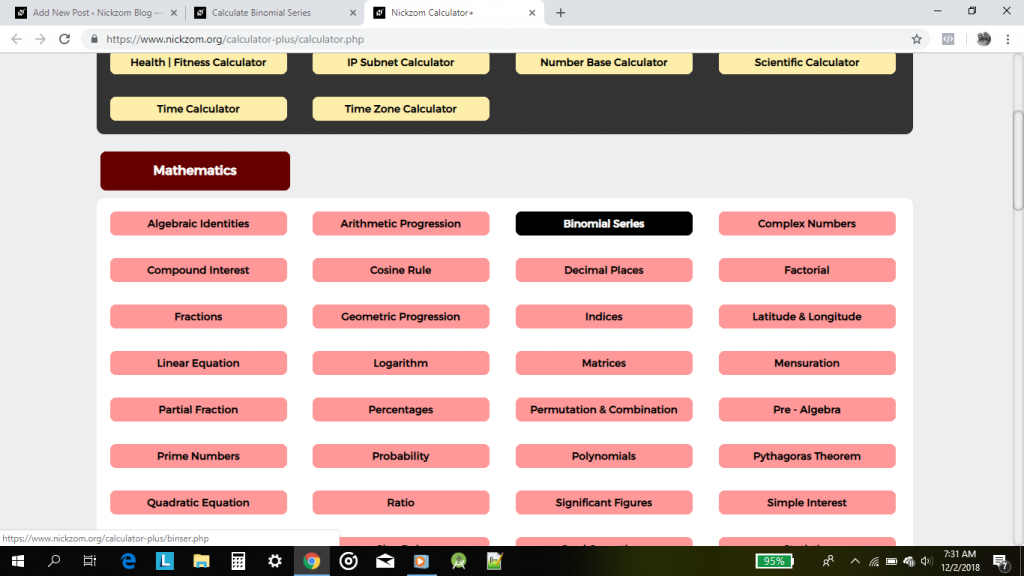For the purpose of this post, let’s compute a binomial series on (2x + 3y)4 , on the binomial series click on (ax + by)nOn clicking, the value entry interface is displayed for you to enter the appropriate values.Now, enter the appropriate values according to the coefficients and parameters.Lastly, click on the Calculate Button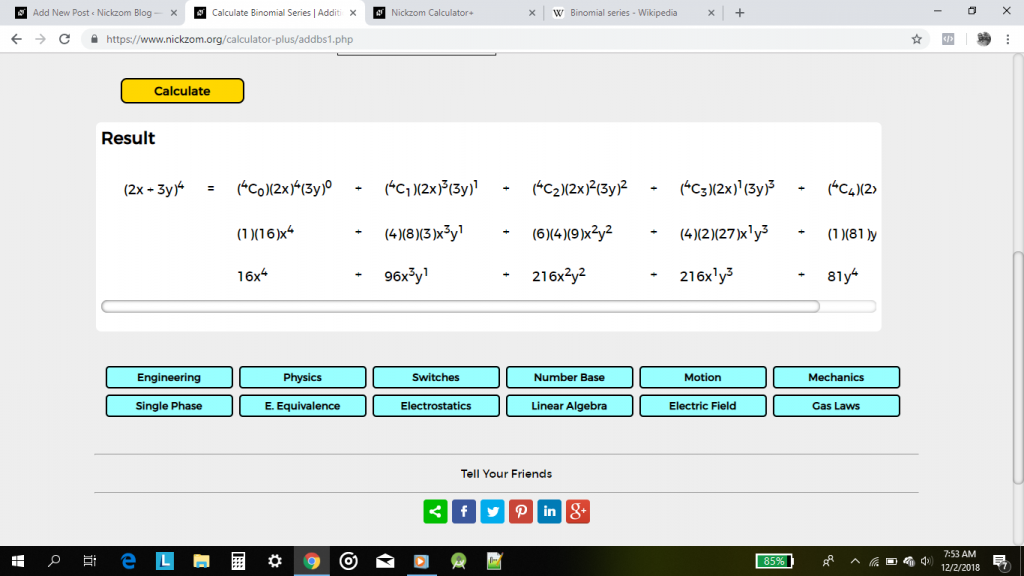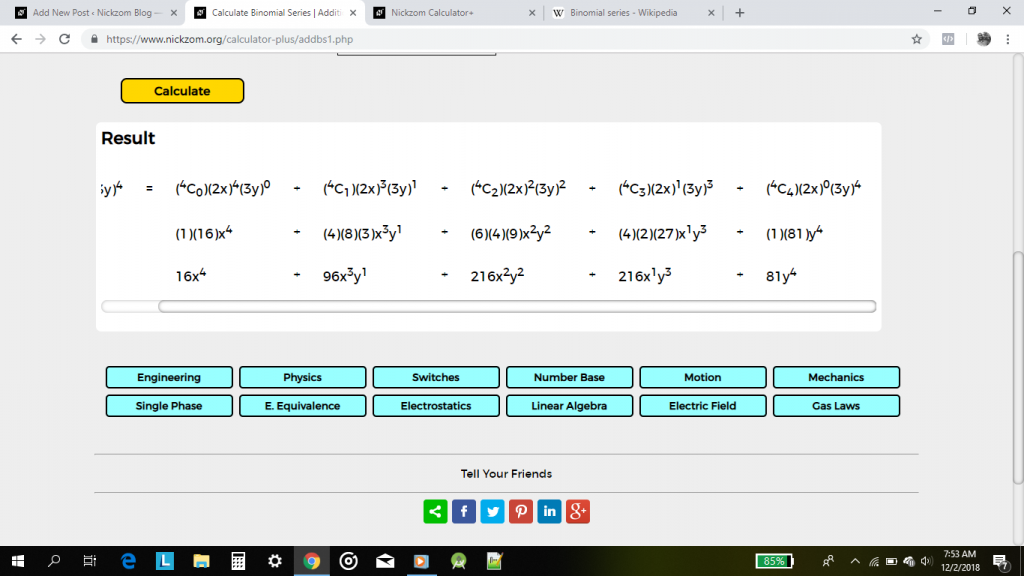Smooth Right? Now, let’s perform this binomial series operation (4 – 5y)3

Proceed back to the Binomial SeriesOn clicking the value entry interface is displayed.Now, enter the values appropriatelyFinally, click on Calculate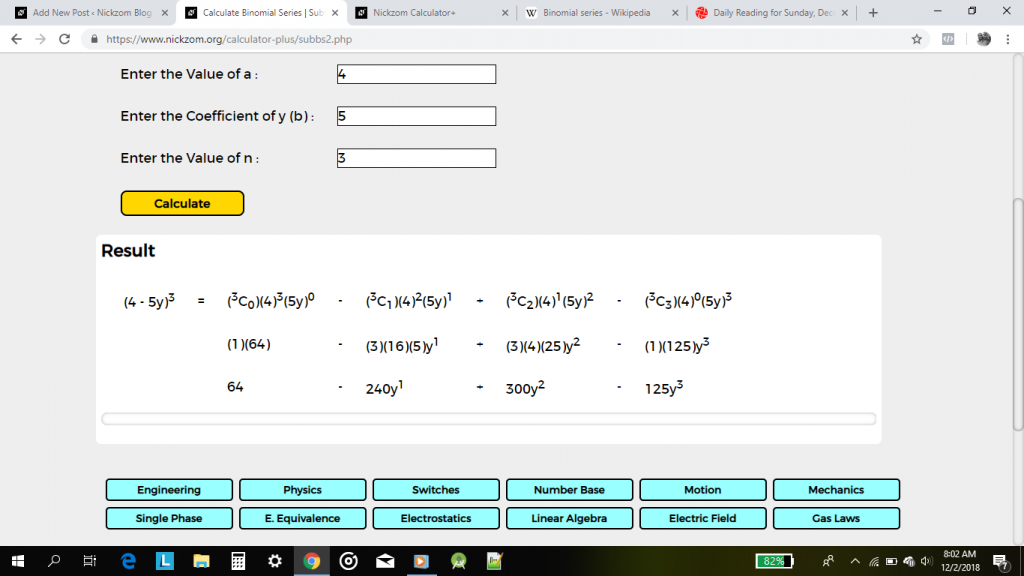The Calculator Encyclopedia currently solves over 50,000 calculations and Binomial Series is one of them. We have the largest repository for getting answers and workings to your calculations and if there is any calculation problem you cannot find in Nickzom Calculator please do let us know for immediate implementation.

You can access Nickzom Calculator (The Calculator Encyclopedia) via any of these channels: